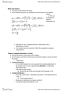# ECO220Y1- Midterm Exam Guide - Comprehensive Notes for the exam ( 14 pages long!)

277 views14 pages
School
Department
Coursejimmm and 37654 others unlocked27
Verified Note
27 documents

## Document Summary

*this course is only about large population statistics, not small population statistics. Key terms: population: set of all items of interest, parameter: number describing population, sample: subset of a population, statistic: number describing a sample, refers exclusively to random samples, can either have a population parameter or a sample statistic. Two branches of statistics: descriptive statistics: describe samples, data, inferential statistics: make inferences about population and its parameters using data. Probability vs statistics: probability looks at a population to decide the likelihood of the composition of a random sample, statistics takes a random sample and tries to determine the population it represents. Sampling variability is technically different, but is used interchangeable with sampling: denotes proportion of a sample that follows a particular characteristic, p denotes proportion of population that follows a particular characteristic. Sampling distribution: how a sample statistic varies because of sampling error. How far off the sample is: bigger populations do not necessitate bigger sample size.

## Get access

\$8 USD/m\$10 USD/m
Billed \$96 USD annuallyHomework Help
Study Guides
Textbook Solutions
Class Notes
Textbook Notes
Booster Class